# Difference Between jQuery Each and Map Method

## Difference Between jQuery Each and Map Method with Examples

In this article, I am going to discuss the Difference Between jQuery Each and Map Method with Examples. Please read our previous article, where we discussed the jQuery Map Method.

##### Difference Between jQuery Each and Map Method

We had a comparative study of the map() and each() method in our previous articles. Now in this article, we will study about the differences between \$.each() and \$.map() in jQuery. The each() method is meant to be an immutable iterator, whereas the map() method can be used as an iterator, but is really meant to manipulate the supplied array and return a new array. The differences between these two functions are as follows.

Syntax of each function: \$.each( function(index, element){})
Syntax of map function: \$.map( function(element, index){})

Notice that, both functions are taking a callback function but the order of the parameters of the callback is different. In each function, the first parameter of the callback is the index of the current element and the second parameter is the current element of the collection. But this is reverse for map function. In the map function, the first parameter of the callback is the current element of the collection and the second parameter is the index of the current element. Let’s see an example to prove that.

```<!DOCTYPE html>
<html>
<title> Difference Between jQuery Each and Map Method</title>
<body>
<script>
let arr = [10, 20, 30, 40, 50];
\$.each(arr, function (index, element) {
console.log(`Index : \${index}   Element : \${element}`);
});
});
</script>
</body>
</html>
```

Now run the above code and open the browser developer tool and have a look at the Console tab. Notice that each function is having parameters index & element as shown in the below image.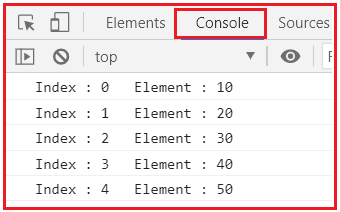Now let’s see for map function.

```<!DOCTYPE html>
<html>
<title> Difference Between jQuery Each and Map Method</title>
<body>
<script>
let arr = [10, 20, 30, 40, 50];
\$.map(arr, function (element, index) {
console.log(`Index : \${index}   Element : \${element}`);
});
});
</script>
</body>
</html>
```

Now run the above code and open the browser developer tool and have a look at the Console tab. Notice that the order of the parameters is different here. Here 2nd parameter is representing the index and the 1st parameter is the value of the current element.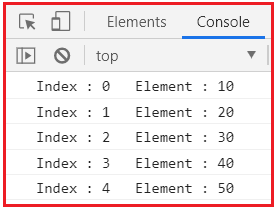Here comes a question. What if we put the parameters in the ‘index, element’ order? Let’s see

```<!DOCTYPE html>
<html>
<title>Difference Between jQuery Each and Map Method</title>
<body>
<script>
let arr = [10, 20, 30, 40, 50];
\$.map(arr, function (index, element) {
console.log(`Index : \${index}   Element : \${element}`);
});
});
</script>
</body>
</html>
```

The answer is clear now. Here whatever may be the name of the 1st parameter that is always receiving the current element of the array, and on the other hand, the 2nd parameter is receiving the index irrespective of the name of the parameter as shown in the below image.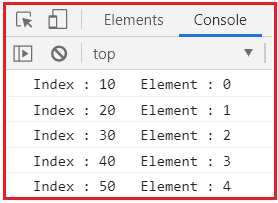##### Return Value in jQuery Each and Map Method:

jQuery each() function is used to perform certain actions on an array by a callback function. It returns the original array and doesn’t change it. On the other hand map() function operates the callback function on the given collection and returns a new array. Let’s understand this difference by an example

```<!DOCTYPE html>
<html>
<title>Difference Between jQuery Each and Map Method</title>
<body>
<script>
let arr = [1, 2, 3, 4, 5];
console.log(
\$.each(arr, function (index, element) {
return element * element;
})
);
});
</script>
</body>
</html>
```

Notice that we are using each function here. But when we are trying to log the return value then it can be seen that it is only the original array that is returning.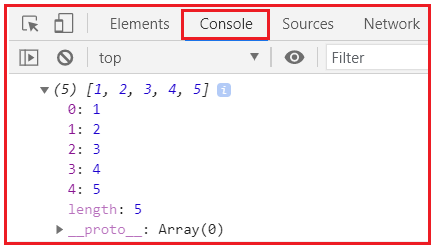Now, look at the map() function.

```<!DOCTYPE html>
<html>
<title>Difference Between jQuery Each and Map Method</title>
<body>
<script>
let arr = [1, 2, 3, 4, 5];
console.log(
\$.map(arr, function (element, index) {
return element * element;
})
);
});
</script>
</body>
</html>
```

The jQuery Map() function performs the operation on each element of the array and is storing those into a new array. In the console, we are getting the new array in which the elements are the square of the elements of the original array.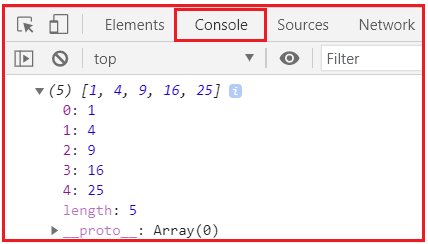##### this keyword in jQuery Each and Map Method:

We know that the ‘this’ keyword refers to the current element or object, over which the iteration is currently going on. But the behavior of the ‘this’ keyword is different for the each() & map() function. Let’s understand these by an example. Look at each function first.

```<!DOCTYPE html>
<html>
<title>Difference Between jQuery Each and Map Method</title>
<body>
<script>
let arr = ["tiger", 20, 30, true, 50];
\$.each(arr, function () {
console.log(this);
});
});
</script>
</body>
</html>
```

Here for every iteration, we are trying to print the current element of the collection on the console by calling the ‘this’ keyword.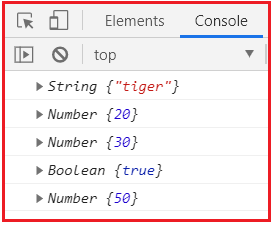Notice that we are getting the current element for every iteration in the console. Now for the map function behavior of the ‘this’ keyword has changed.

```<!DOCTYPE html>
<html>
<title>Difference Between jQuery Each and Map Method</title>
<body>
<script>
let arr = ["tiger", 20, 30, true, 50];
\$.map(arr, function () {
console.log(this);
});
});
</script>
</body>
</html>
```

Here ‘this’ keyword is not referring to the current element. Here ‘this’ is just referring to the window object.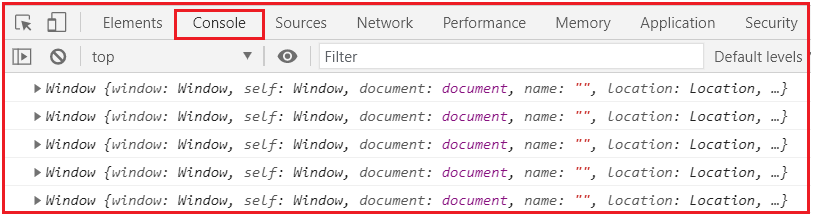##### Terminating the iteration in jQuery Each and Map Method:

We have a way to terminate the iteration in each() function. But there is no way to terminate the iteration in the map() method. Let’s see by example.

```<!DOCTYPE html>
<html>
<title>Difference Between jQuery Each and Map Method</title>
<body>
<script>
let arr = [10, 20, 30, 40, 50];
\$.each(arr, function (index, element) {
if (element == 30) {
return false;
}
console.log(`Index : \${index}  Element : \${element}`);
});
});
</script>
</body>
</html>
```

We use return false; at any stage of the iteration to break the loop. Here we are telling our program that if the current element equal to 30 then the loop should be broken and iteration should not be executed for the rest of the elements. Notice that in the console we are getting the only result of the first two iterations.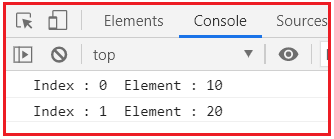Now, look at the map method.

```<!DOCTYPE html>
<html>
<title>Difference Between jQuery Each and Map Method</title>
<body>
<script>
let arr = [10, 20, 30, 40, 50];
\$.map(arr, function (element, index) {
if (element == 30) {
return false;
}
console.log(`Index : \${index}  Element : \${element}`);
});
});
</script>
</body>
</html>
```

Here we are using the return false; in the same way but the behavior is different here. So here what is happening? When the iteration reaches the if the block at (element == 30) and checks the condition is true, it is returning false, but the iteration doesn’t stop. That one element is not returned but the loop continues and for the rest two elements the code is executing and performing the function. So, we are getting all elements except 30. In this way, we can remove an element from a collection while using the map function.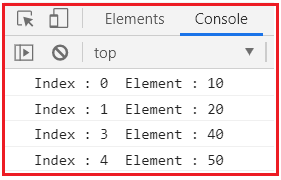##### Performance in jQuery Each and Map Method:

Let’s take a look at which method takes more time.

```<!DOCTYPE html>
<html>
<title>Difference Between jQuery Each and Map Method</title>
<body>
<script>
let arr = [10, 20, 30, 40, 50];
var startEach = performance.now();
\$.each(arr, function (index, element) {
console.log(index + element * 10000000000);
});
var endEach = performance.now();
console.warn(`Time taken by each() : \${endEach - startEach} ms`);

var startMap = performance.now();
\$.map(arr, function (element, index) {
console.log(index + element * 10000000000);
});
var endMap = performance.now();
console.warn(`Time taken by map() : \${endMap - startMap} ms`);
});
</script>
</body>
</html>
```

We are measuring the time taken by the function to call by JavaScript performance.now() method.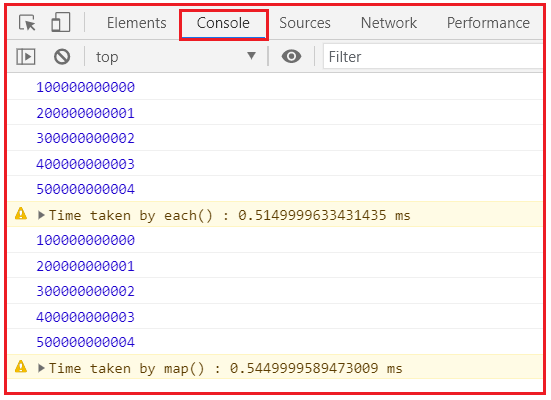You can see that there is a little difference in the performance time of the two functions. But that is not conclusive that may change for different parameters.

So, we can conclude that each and map function is based on your use case. If you want to create a new array that can be used for further methods like reduce(), filter() then you should go for the map function. If you only wish to perform any action to the elements of your collection then opt for each method.

In the next article, I am going to discuss the jQuery Change Event with Examples. Here, in this article, I try to explain the difference between Each and Map in jQuery with Examples and I hope you enjoy this Difference between Each and Map in the jQuery article.

### 1 thought on “Difference Between jQuery Each and Map Method”

1.Nice, thank´s !